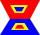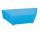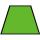# Length + trapezoid - math problems

#### Number of problems found: 57

• Trapezoid 25Trapezoid PART with AR||PT has (angle P=x) and (angle A=2x) . In addition, PA = AR = RT = s. Find the length of the median of Trapezoid PART in terms of s.
• A trapezoidA trapezoid with a base length of a = 36.6 cm, with angles α = 60°, β = 48° and the height of the trapezoid is 20 cm. Calculate the lengths of the other sides of the trapezoid.
• Rectangular trapezoidThe rectangular trapezoid ABCD is: /AB/ = /BC/ = /AC/. The length of the median is 6 cm. Calculate the circumference and area of a trapezoid.
• Isosceles trapezoidFind the area of an isosceles trapezoid with bases of 8cm and 72mm. The height of the trapezoid is equal to three-quarters of the longer base.
• Length ITFind the length (circumference) of an isosceles trapezoid in which the length of the bases a,c, and the height h are given: a = 8 cm c = 2 cm h = 4 cm.
• Trapezoid thirdsThe ABCD trapezoid with the parallel sides of the AB and the CD and the E point of the AB side. The segment DE divides the trapezoid into two parts with the same area. Find the length of the AE line segment.
• Area of trapezoidThe trapezoid bases are and 7 dm and 11 cm. His height is 4 cm. Calculate the area of the trapezoid.
• Right trapezoidThe right trapezoid has bases 3.2 cm and 62 mm long. The shorter leg has a length 0.25 dm. Calculate the lengths of the diagonals and the second leg.
• BasesThe length of the bases trapezium are in ratio 4:5. Length of midline is 15. How long are the bases of a trapezoid?
• Four sides of trapezoidTrapezoid is given by length of four sides: 40.5 42.5 52.8 35.0. Calculate its area.
• Trapezoid ABCD v2Trapezoid ABCD has a length of bases in ratio 3:10. The area of triangle ACD is 825 dm2. What is the area of trapezoid ABCD?
• Trapezoid ABCDABDC is a trapezoid in which AB and CD are parallel sides measuring 6 and 9, respectively. Angles ABC and BCD are both right angles. Find the length of segment BD.
• Isosceles trapezoidThe lengths of the bases of the isosceles trapezoid are in the ratio 5:3, the arms have a length of 5 cm and height = 4.8 cm. Calculate the circumference and area of a trapezoid.
• Trapezoid - diagonalA trapezoid has a length of diagonal AC crossed with diagonal BD in the ratio 2:1. The triangle created by points A, cross point of diagonals S and point D has area 164 cm2. What is the area of the trapezoid?
• The ABCDThe ABCD trapezoid has a base length of a = 120mm, c = 86mm and an area of S = 2575 mm2. Calculate the height of the trapezoid.
• Drainage channelThe cross section of the drainage channel is an isosceles trapezoid whose bases have a length of 1.80 m, 0.90 m and arm has length 0.60 meters. Calculate the depth of the channel.
• Isosceles trapezoidFind the area of an isosceles trapezoid, if the bases are 12 cm and 20 cm, the length of the arm is 16 cm
• IS trapezoidIsosceles trapezoid arm measured 35 cm. Height is 30 cm and middle segment is 65 cm. Determine length of its bases.
• The rectangularThe rectangular trapezoid has bases 15 dm and 8 dm long and the length of the inclined arm is 12 dm. How long is the other arm of the trapezoid?
• Children playgroundThe playground has a trapezoid shape, and the parallel sides have a length of 36 m and 21 m. The remaining two sides are 14 m long and 16 m long. Find the size of the inner trapezoid angles.

Do you have an interesting mathematical word problem that you can't solve it? Submit a math problem, and we can try to solve it.

We will send a solution to your e-mail address. Solved examples are also published here. Please enter the e-mail correctly and check whether you don't have a full mailbox.

Please do not submit problems from current active competitions such as Mathematical Olympiad, correspondence seminars etc...

Do you want to convert length units? Length - math problems. Trapezoid Problems.